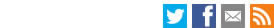Search the LSE websiteAbstract:CEP Discussion Paper
How to Measure Living Standards and Productivity
Nicholas Oulton
September 2009
Paper No' CEPDP0949:
Full PaperJEL Classification: C43; D11; D12; E31; D24; I31; O47

This paper sets out a general algorithm for calculating true cost-of-living indices or true producer price indices when demand is not homothetic, i.e. when not all expenditure elasticities are equal to one. In principle, economic theory tells us how we should calculate a true cost-of-living index or Konüs price index: first estimate the consumer’s expenditure function (cost function) econometrically and then calculate the Konüs price index directly from that. Unfortunately this is impossible in practice since real life consumer (producer) price indices contain hundreds of components, which means that there are many more parameters than observations. Index number theory has solved this problem, at least when demand is homothetic (all income elasticities equal to one). Superlative index numbers are second order approximations to any acceptable expenditure (cost) function. These index numbers require data only on prices and quantities over the time period or cross section under study. Unfortunately, there is overwhelming evidence that consumer demand is not homothetic (Engel’s Law). The purpose of the present paper is to set out a general algorithm for the nonhomothetic case. The solution is to construct a chain index number using compensated, not actual, expenditure shares as weights. The compensated shares are the actual shares, adjusted for changes in real income. These adjustments are made via an econometric model, where only the responses of demand to income changes need to be estimated, not the responses to price changes. This makes the algorithm perfectly feasible in practice. The new algorithm can be applied (a) in time series, e.g. measuring changes over time in the cost of living; (b) in cross section, e.g. measuring differences in the cost of living and hence the standard of living across countries; and (c) to cost functions, which enables better measures of technical progress to be developed.